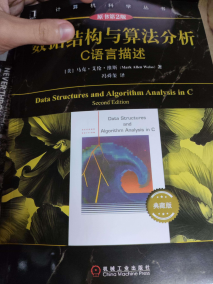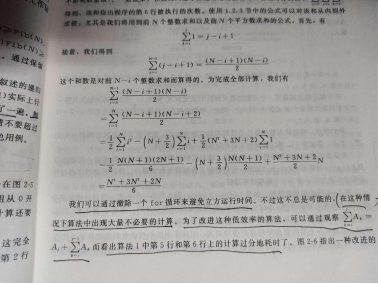# 3月1日至3月2日——数据结构与算法分析阅读笔记，线性表，AI。

（开头是一些废话啊，最近感觉学习状态不太好，上高数的时候左耳听进去右耳就出来了，有点跟不上，可能是没吃饭的原因，也可能是最近强度有点大了，下午上完课就给自己休息了一下，结果刷手机全是关于AI的内容，谢谢你，大数据。躺了两个小时感觉状态也不是太好。）============================================================================================================

long int
Fib( int N)
{//斐波那契数
if( N<=1 )//第一行
return 1;//第二行
else
return Fib( N - 1 ) + Fib( N - 2);//第三行（感觉写注释确实挺烦的）
}


int Max( const int A[],int N)
{
int ThisSum,MaxSum,i,j,k;

MaxSum = 0;//第一行
for( i = 0; i < N; i++ )//第二行
for( j = i; j < N; j++ )//第三行
{
ThisSum = 0;//第四行
for( k = i; k <= j; k++ )//第五行
ThisSum += A[k];//第六行

if( ThisSum > MaxSum )//第七行
MaxSum = ThisSum;//第八行
}
return MaxSum;//第九行
}int Max( const int A[],int N)
{
int ThisSum,MaxSum,i,j,k;

MaxSum = 0;//第一行
for( i = 0; i < N; i++ )//第二行
{
ThisSum = 0;//第三行
for( j = i; j < N; j++ )//第四行
{
ThisSum += A[j];//第五行

if( ThisSum > MaxSum )//第六行
MaxSum = ThisSum;//第七行
}
}
return MaxSum;//第八行
}


AI（挺重要的，可以看一下吗）posted @ 2023-03-02 18:43  apeiriaDolce  阅读(11)  评论(0编辑  收藏  举报